Contemporary Mathematics

# 5.5Graphing Linear Equations and Inequalities

Contemporary Mathematics5.5 Graphing Linear Equations and Inequalities

Figure 5.18 How much would it cost to fill up your gas tank? (credit: "Gas Under 4 Bucks" by Mark Turnauckas, Flickr/CC BY 2.0)

### Learning Objectives

After completing this section, you should be able to:

1. Graph linear equations and inequalities in two variables.
2. Solve applications of linear equations and inequalities.

1.
If a stamp costs $0.55 and you buy a book of 20 stamps, then you pay$11. If you want to mail 100 letters, you can buy a roll of stamps for $55. Your friend only needs 3 stamps, how much will they pay? ### People in Mathematics #### René Descartes Figure 5.28 René Descartes (credit: Flickr, Public Domain) René Descartes was born in 1596 in La Haye, France. He was sickly as a child, so much so that he was allowed to stay in bed until 11:00 AM rather than get up at 5:00 AM like the other school children. He kept this habit of rising late for most of the rest of his life. After his primary schooling, Descartes attended the University of Poitiers, receiving a law degree in 1616. He then embarked on a myriad of journeys, joining two different militaries (one in the Netherlands, the other in Bavaria) and generally travelling around Europe until 1628, when he settled in the Netherlands. It was here that he began to delve deeply into his ideas of science, mathematics, and philosophy. In 1637, at the urging of his friends, Descartes published Discourse on the Method for Conducting One's Reason Well and Seeking the Truth in the Sciences. The book had three appendices: La Dioptrique, a work on optics; Les Météores, which pertained to meteorology; and La Géométrie, a work on mathematics. It was in this appendix that he proposed a geometric way of representing many different algebraic expressions and equations. It is this system of representation that almost all mathematical textbooks use today. These publications (along with several others) brought much fame to Descartes. So renowned was his reputation that late in 1649, Queen Christina of Sweden asked Descartes to come to Sweden to tutor her. However, she wished to do her studies at 5:00 in the morning; Descartes had to break his lifelong habit of sleeping in late. A few months later, in February 1650, Descartes died of pneumonia. ### Graphing Linear Inequalities Previously we learned to solve inequalities with only one variable. We will now learn about inequalities containing two variables that can be written in one of the following forms: $Ax+By≥CAx+By≥C$, $Ax+By>CAx+By>C$, $Ax+By≤CAx+By≤C$, and $Ax+By where $AA$ and $BB$ are not both zero. We will look at linear inequalities in two variables, which are very similar to linear equations in two variables. Like linear equations, linear inequalities in two variables have many solutions. Any ordered pair ($xx$, $yy$) that makes an inequality true when we substitute in the values is a solution to a linear inequality. ### Example 5.43 #### Determining Solutions to an Inequality Determine whether each ordered pair is a solution to the inequality $y>x+4y>x+4$: 1. $(0,0)(0,0)$ 2. $(1,6)(1,6)$ 3. $(2,6)(2,6)$ 4. $(–5,–15)(–5,–15)$ 5. $(−8,12)(−8,12)$ ### Your Turn 5.43 Determine whether each ordered pair is a solution to the inequality $y > x - 1{:}$ 1. $(0,1)$ 2. $( - 4, - 1)$ 3. $(4,2)$ 4. $(3,0)$ 5. $( - 2, - 3)$ Let us think about $x>3x>3$. The point $x=3x=3$ separated that number line into two parts. On one side of 3 are all the numbers less than 3. On the other side of 3 all the numbers are greater than 3. See Figure 5.29. Figure 5.29 Solution to $x>3x>3$ on a Number Line Similarly, the line $y=x+4y=x+4$ separates the plane into two regions. On one side of the line are points with $y. On the other side of the line are the points with $y>x+4y>x+4$. We call the line $y=x+4y=x+4$ a boundary line. For an inequality in one variable, the endpoint is shown with a parenthesis (Figure 5.30) or a bracket (Figure 5.31) depending on whether or not $aa$ is included in the solution: Figure 5.30 Endpoint with Parenthesis Figure 5.31 Endpoint with Bracket Similarly, for an inequality in two variables, the boundary line is shown with a solid or dashed line to show whether or not it the line is included in the solution.  $Ax+By $Ax+By≤CAx+By≤C$ $Ax+By>CAx+By>C$ $Ax+By≥CAx+By≥C$ Boundary line is $Ax+By=CAx+By=C$ Boundary line is $Ax+By=CAx+By=C$ Boundary line is not included in solution. Boundary line is included in solution. Boundary line is dashed. Boundary line is solid. Now, let us take a look at what we found in Example 5.43. We will start by graphing the line $y=x+4y=x+4$, and then we will plot the five points we tested, as graphed in Figure 5.32. We found that some of the points were solutions to the inequality $y>x+4y>x+4$ and some were not. Which of the points we plotted are solutions to the inequality $y>x+4y>x+4$? The points $(1,6)(1,6)$ and $(−8,12)(−8,12)$ are solutions to the inequality $y>x+4y>x+4$. Notice that they are both on the same side of the boundary line $y=x+4y=x+4$. The two points $(0,0)(0,0)$ and $(−5,−15)(−5,−15)$ are on the other side of the boundary line $y=x+4y=x+4$, and they are not solutions to the inequality $y>x+4y>x+4$. For those two points, $y. What about the point $(2,6)(2,6)$? Because $6=2+46=2+4$, the point is a solution to the equation $y=x+4y=x+4$, but not a solution to the inequality $y>x+4y>x+4$. So, the point $(2,6)(2,6)$ is on the boundary line. Figure 5.32 Graph of $y=x+4y=x+4$ Let us take another point above the boundary line and test whether or not it is a solution to the inequality $y>x+4y>x+4$. The point $(0,10)(0,10)$ clearly looks to be above the boundary line, doesn’t it? Is it a solution to the inequality? $y>x+410>?0+410>4✓y>x+410>?0+410>4✓$ Yes, $(0,10)(0,10)$ is a solution to $y>x+4y>x+4$. Any point you choose above the boundary line is a solution to the inequality $y>x+4y>x+4$. All points above the boundary line are solutions. Similarly, all points below the boundary line, the side with $(0,0)(0,0)$ and $(−5,−15)(−5,−15)$, are not solutions to $y>x+4y>x+4$, as shown in Figure 5.33. Figure 5.33 Graph of $y=x+4y=x+4$, with $y>x+4y>x+4$ Above the Boundary Line and $y Below the Boundary Line The graph of the inequality $y>x+4y>x+4$ is shown in Figure 5.34. The line $y=x+4y=x+4$ divides the plane into two regions. The shaded side shows the solutions to the inequality $y>x+4y>x+4$. The points on the boundary line, those where $y=x+4y=x+4$, are not solutions to the inequality $y>x+4y>x+4$, so the line itself is not part of the solution. We show that by making the boundary line dashed, not solid. Figure 5.34 Graph of $y>x+4y>x+4$ ### Example 5.44 #### Writing a Linear Inequality Shown by a Graph The boundary line shown in this graph is $y=2x−1y=2x−1$. Write the inequality shown in Figure 5.35. Figure 5.35 ### Your Turn 5.44 1. Write the inequality shown by the graph with the boundary line $y = -2x + 3$.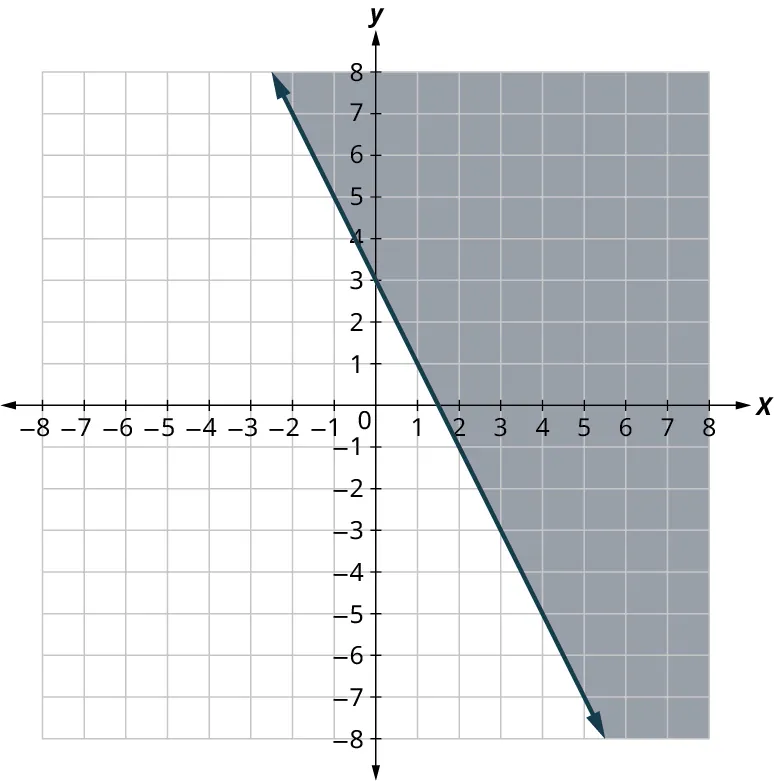### Example 5.45 #### Graphing a Linear Inequality Graph the linear inequality $y≥34x−2y≥34x−2$. ### Your Turn 5.45 1. Graph the linear inequality: $y\, > \,\frac{2x}{3} - 1$. ### Video ### Solving Applications Using Linear Inequalities in Two Variables Many fields use linear inequalities to model a problem. While our examples may be about simple situations, they give us an opportunity to build our skills and to get a feel for how they might be used. ### Example 5.46 #### Working Multiple Jobs Hilaria works two part time jobs to earn enough money to meet her obligations of at least$240 a week. Her job in food service pays $10 an hour and her tutoring job on campus pays$15 an hour. How many hours does Hilaria need to work at each job to earn at least $240? 1. Let $xx$ be the number of hours she works at the job in food service and let $yy$ be the number of hours she works tutoring. Write an inequality that would model this situation. 2. Graph the inequality. 3. Find three ordered pairs ($x,yx,y$) that would be solutions to the inequality. Then, explain what that means for Hilaria. ### Your Turn 5.46 Harrison works two part time jobs. One at a gas station that pays$11 an hour and the other is as an IT consultant for $16.50 an hour. Between the two jobs, Harrison wants to earn at least$330 a week. How many hours does Harrison need to work at each job to earn at least \$330?
1.
Let $x$ be the number of hours he works at the gas station and let $y$ be the number of hours he works as an IT consultant. Write an inequality that would model this situation.
2.
Graph the inequality.
3.
Find three ordered pairs ($x$, $y$) that would be solutions to the inequality. Then, explain what that means for Harrison.

43.
Choose the correct solution to the equation $6y + 10 = 12y$.
1. $y = 5$
2. $y = -1$
3. $y = \frac{1}{2}$
4. $y = \frac{5}{3}$
44.
Choose the correct graph for $y = 3x + 5$.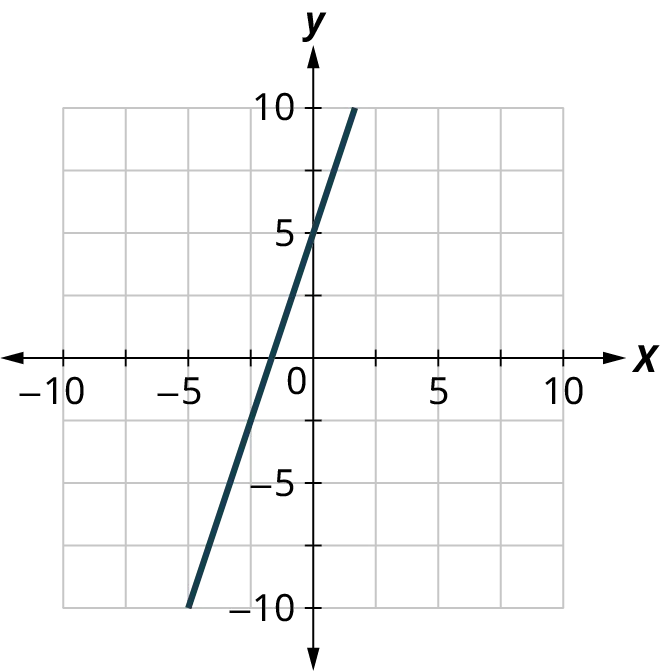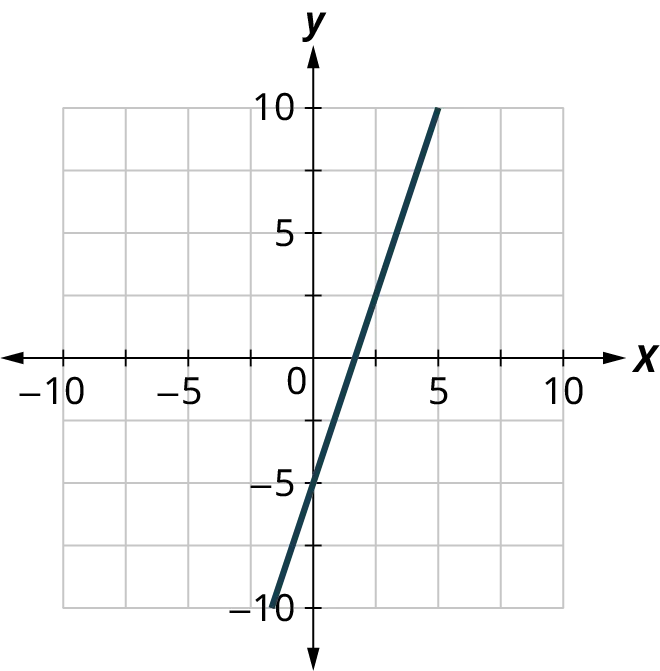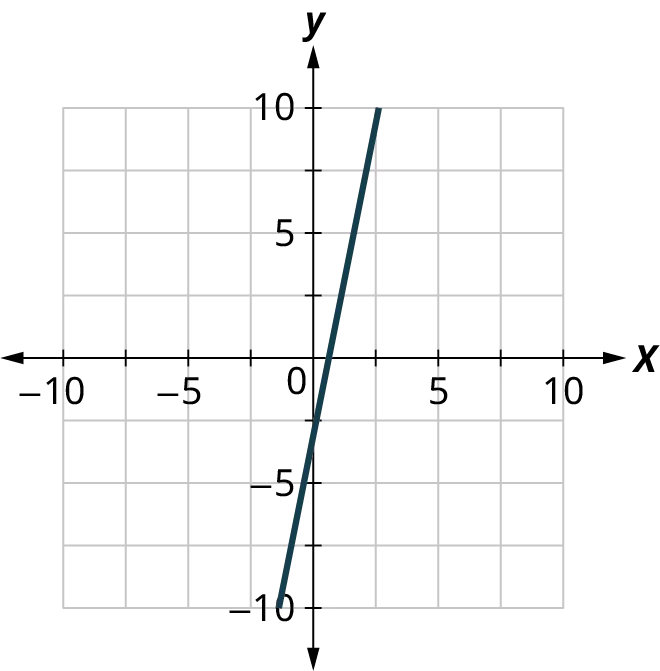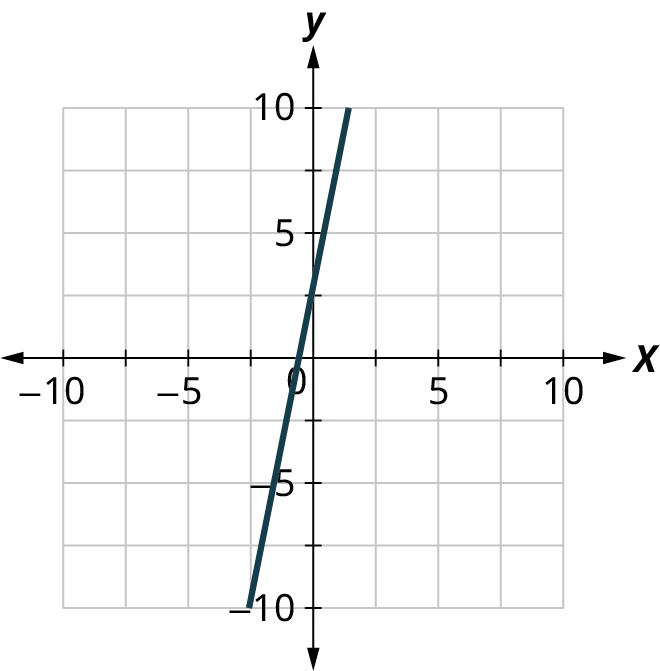45.
Choose the correct equation for the graph shown: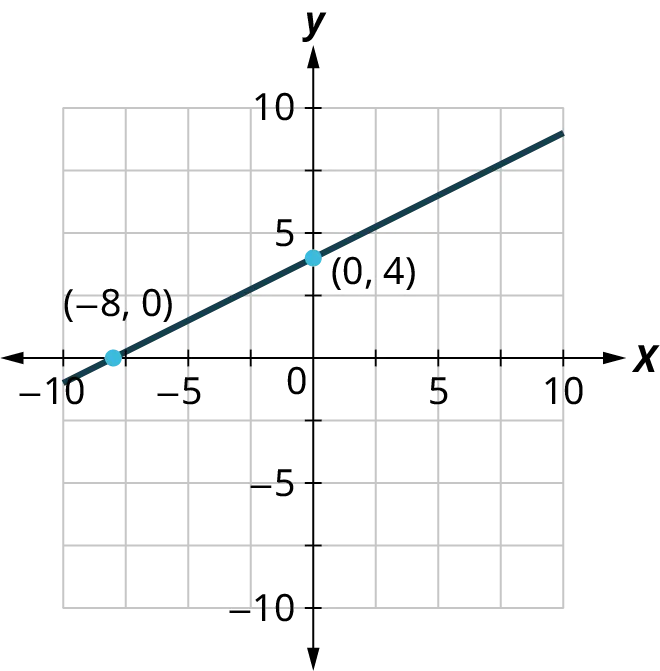1. $y = 2x + 4$
2. $y = \frac{1}{2}x + 4$
3. $y = - 2x + 4$
4. $y = \frac{1}{2}x + 4$
46.
Choose the correct graph for $y > 3x + 5$.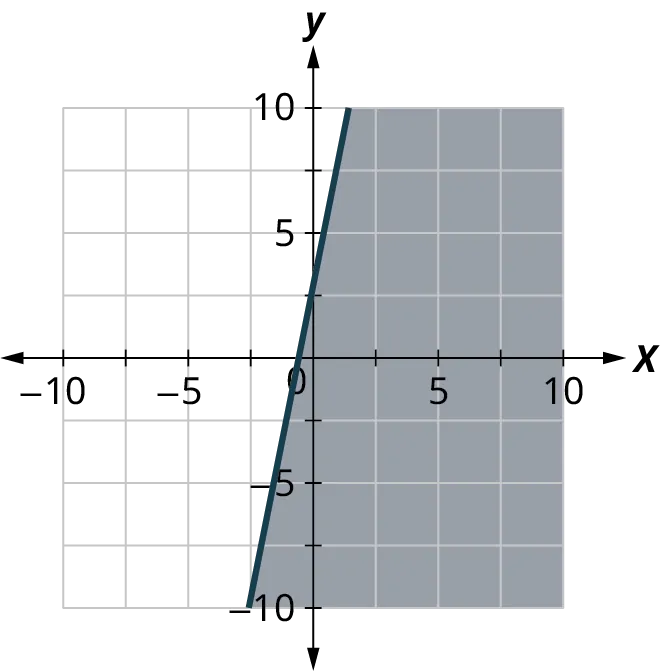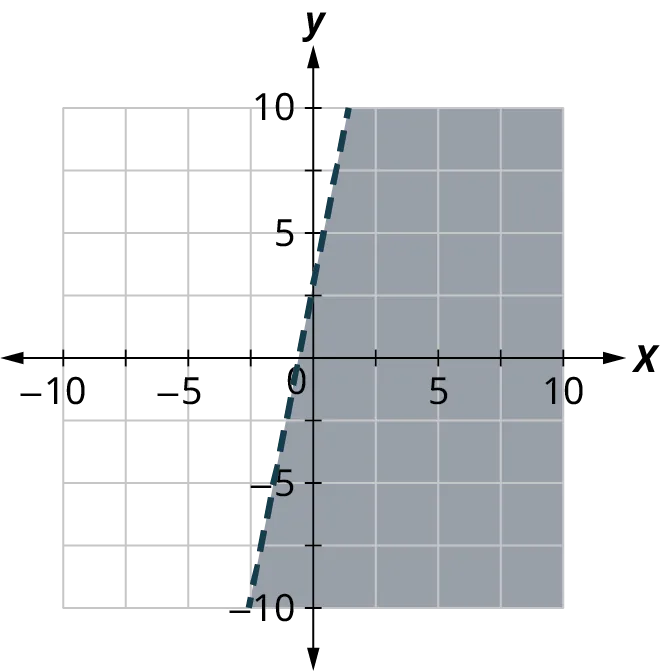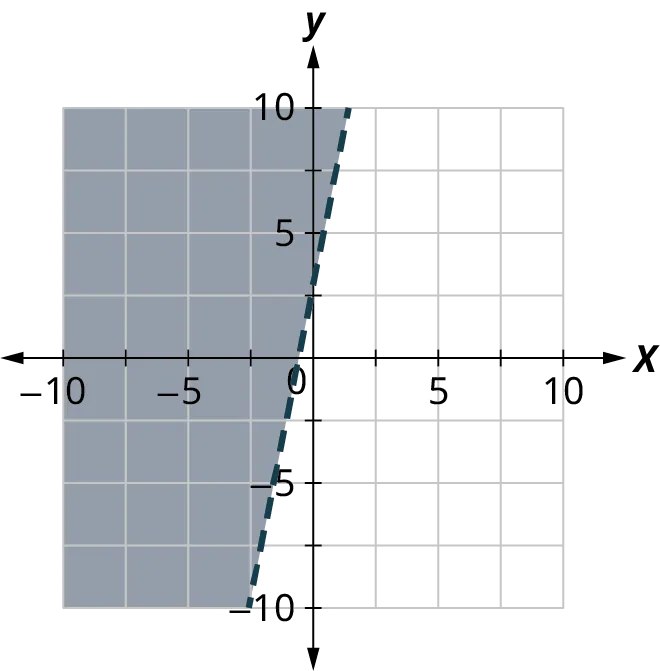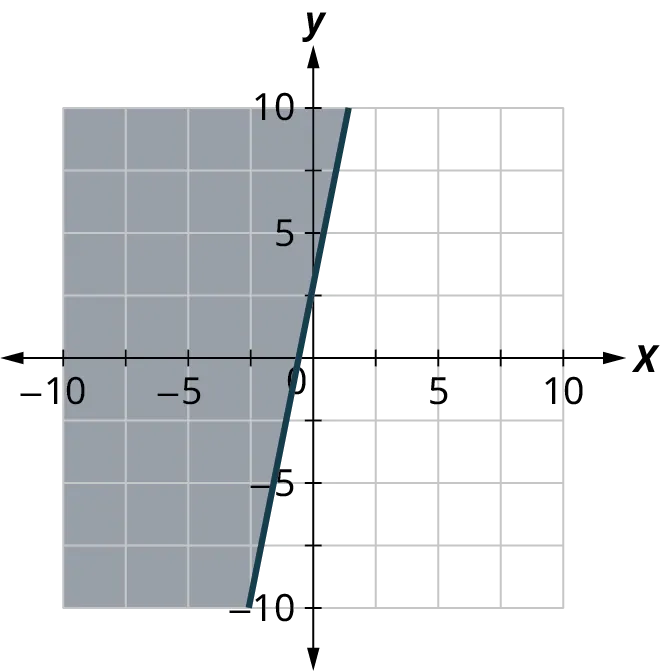47.
Choose the correct inequality for the graph shown.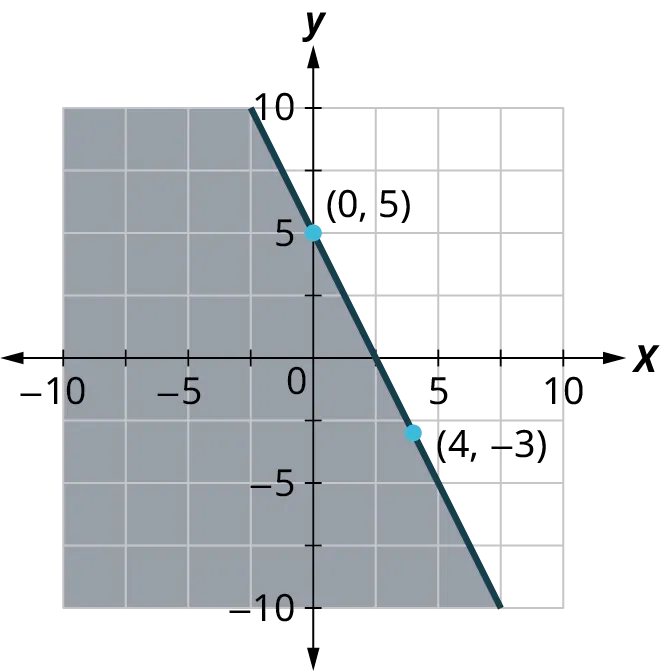1. $y = -2x + 5$
2. $y \leq -2x + 5$
3. $y \geq -2x + 5$
4. $y < -2x + 5$

### Section 5.5 Exercises

1 .
Plot each point in a rectangular coordinate system and identify the quadrant in which the point is located.
1. $(3, -1)$
2. $(-3, 1)$
3. $(-2, 0)$
4. $(-4, -3)$
5. $(1,\frac{{14}}{5})$
For each ordered pair below, decide:
1. Is the ordered pair a solution to the equation?
2. Is the point on the line in the given graph?
$y = \frac{1}{2}x - 3$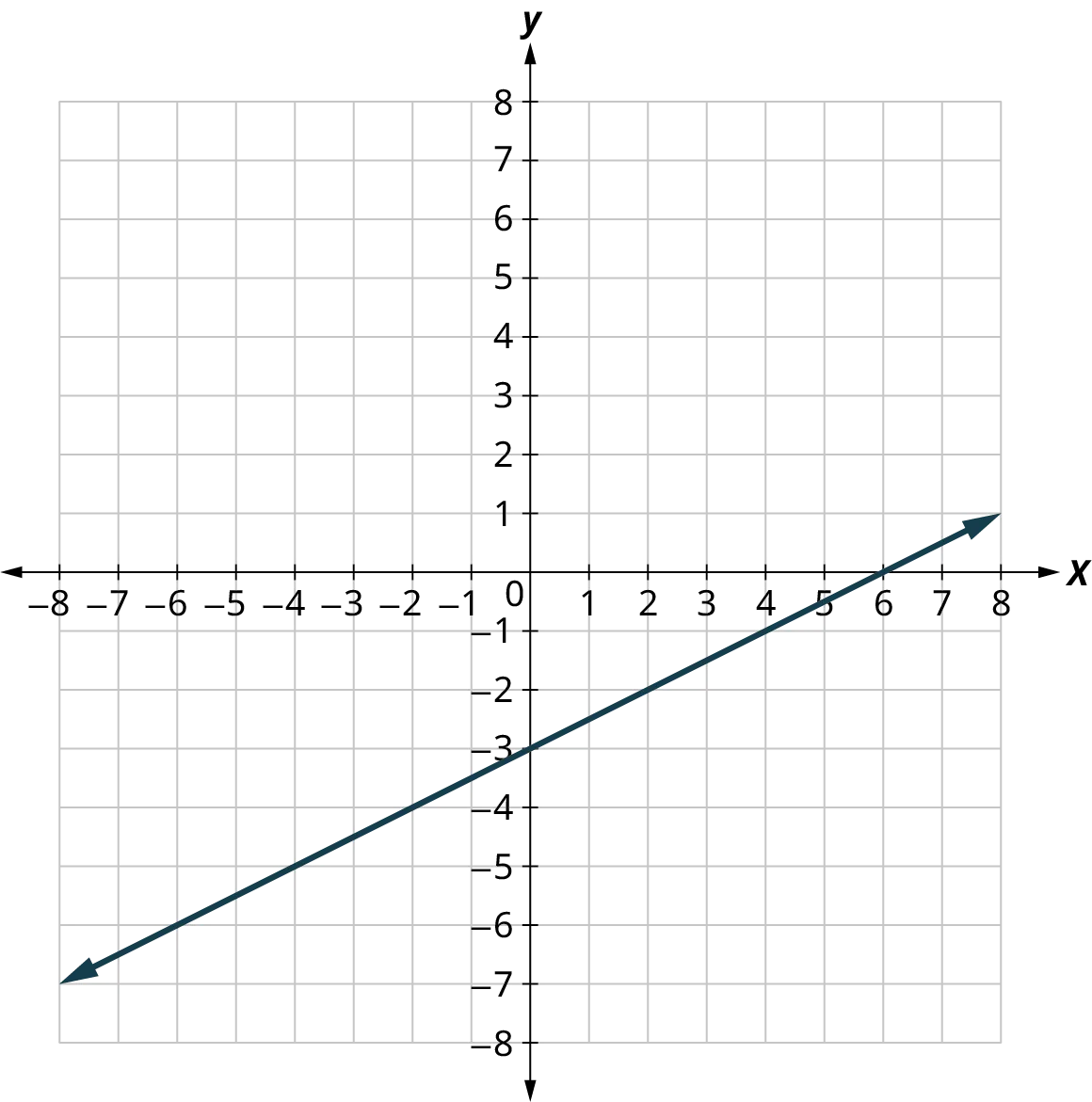2 .
$\left( {0,-3} \right)$
3 .
$\left( {2,-2} \right)$
4 .
$\left( {-2,-4} \right)$
5 .
$\left( {4,1} \right)$
For each ordered pair below, decide:
1. Is the ordered pair a solution to the equation?
2. Is the point on the line in the given graph?
$y = x - 4$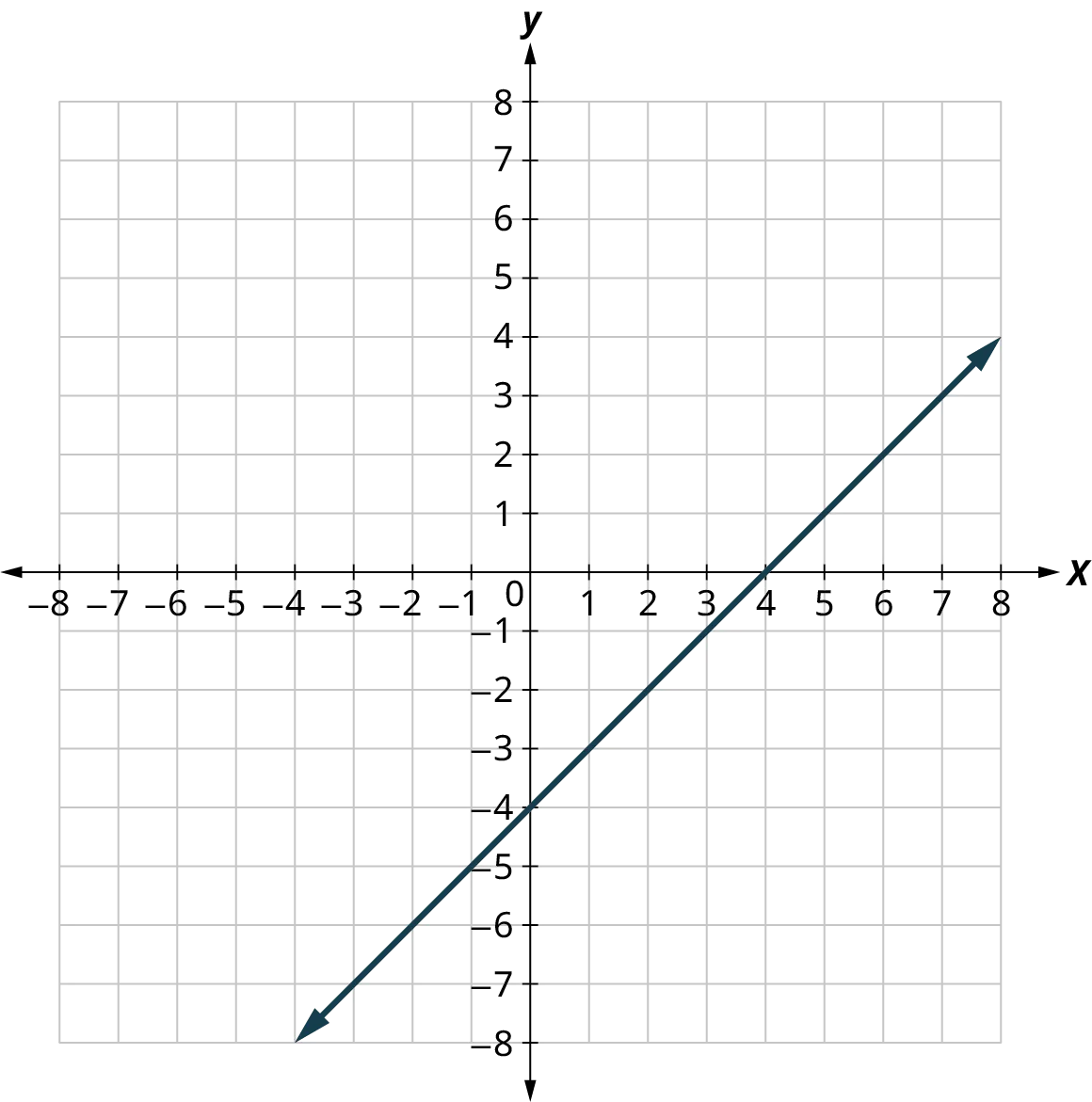6 .
$\left( {0,-4} \right)$
7 .
$\left( {3,-1} \right)$
8 .
$\left( {2,2} \right)$
9 .
$\left( {1,-5} \right)$
For each ordered pair below, decide:
1. Is the ordered pair a solution to the equation?
2. Is the point on the line in the given graph?
$y = \frac{1}{3}x + 2$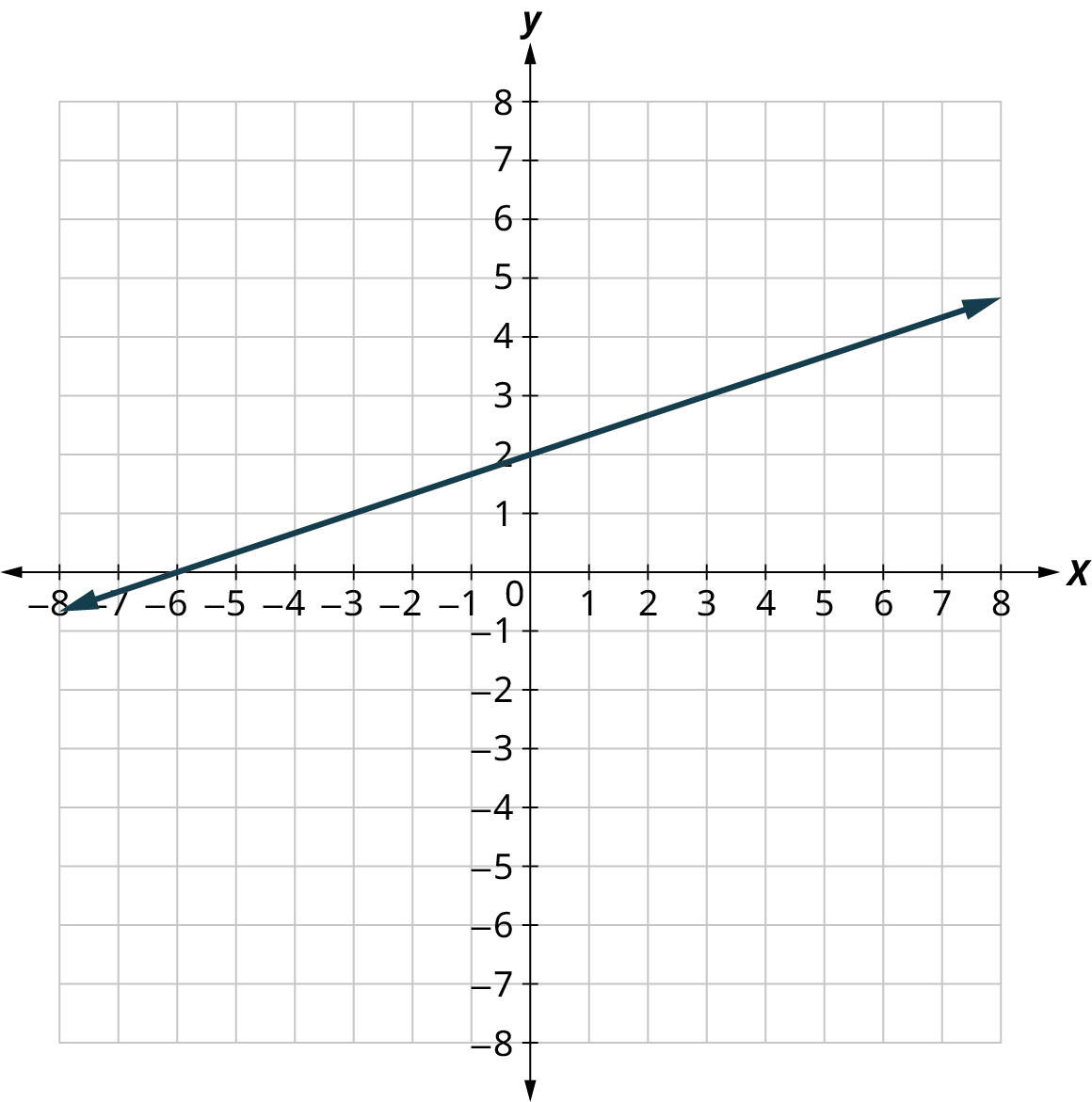10 .
$\left( {0,2} \right)$
11 .
$\left( {3,3} \right)$
12 .
$\left( {-3,2} \right)$
13 .
$\left( {-6,0} \right)$
For the following exercises, graph by plotting points.
14 .
$y = 3x - 1$
15 .
$y = -x - 3$
16 .
$y = 2x$
17 .
$y = \frac{1}{2}x + 2$
18 .
$y = \frac{4}{3}x - 5$
19 .
$y = \frac{2}{5}x + 1$
20 .
$y = \frac{3}{2}x + 2$
For the following exercises, determine whether each ordered pair is a solution to the inequality.
21 .
$y > x-3$
${\text{A: (0, 0)}}\quad\quad{{\text{B: (2, 1)}}}\quad\quad{{\text{C: (-1, -5)}}}\quad\quad{{\text{D: (-6, -3)}}}\quad\quad{{\text{E: (1, 0)}}}$
22 .
$y < 3x + 2$
${\text{A: (0, 3)}}\quad\quad{{\text{B: (-3, -2)}}}\quad\quad{{\text{C: (-2, 0)}}}\quad\quad{{\text{D: (0, 0)}}}\quad\quad{{\text{E: (-1, 4)}}}$
23 .
$y < -2x + 5$
${\text{A: (-3, 0)}}\quad\quad{{\text{B: (1, 6)}}}\quad\quad{{\text{C: (-6, -2)}}}\quad\quad{{\text{D: (0, 1)}}}\quad\quad{{\text{E: (5, -4)}}}$
24 .
$3x - 4y > 4$
${\text{A: (5, 1)}}\quad\quad{{\text{B: (-2, 6)}}}\quad\quad{{\text{C: (3, 2)}}}\quad\quad{{\text{D: (10, -5)}}}\quad\quad{{\text{E: (0, 0)}}}$
25 .
$2x + 3y > 2$
${\text{A: (1, 1)}}\quad\quad{{\text{B: (4, -3)}}}\quad\quad{{\text{C: (0, 0)}}}\quad\quad{{\text{D: (-8, 12)}}}\quad\quad{{\text{E: (3, 0)}}}$
26 .
Write the inequality shown by the graph with the boundary line $y = 3x - 4$.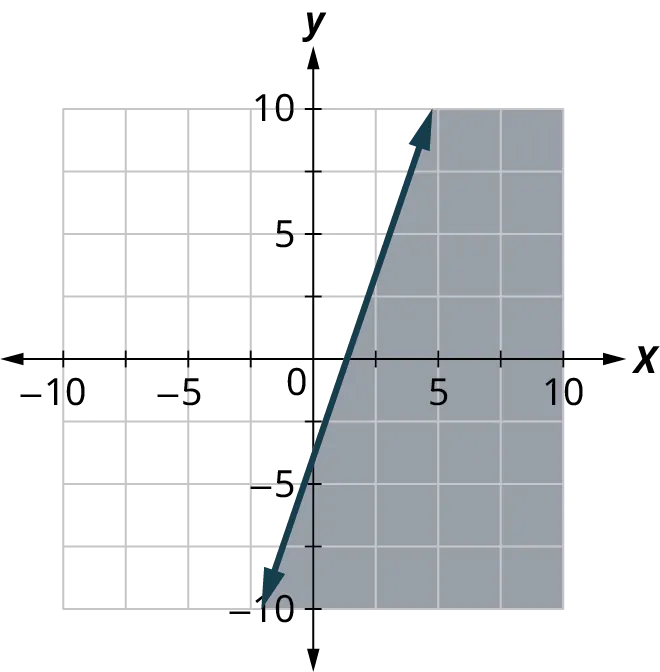27 .
Write the inequality shown by the graph with the boundary line $y = 2x - 4$.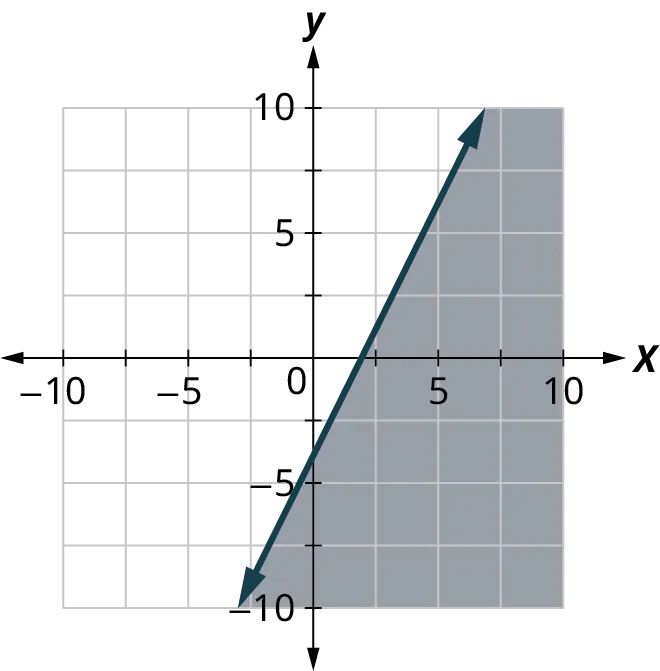28 .
Write the inequality shown by the graph with the boundary line $y = \frac{1}{2}x + 1$.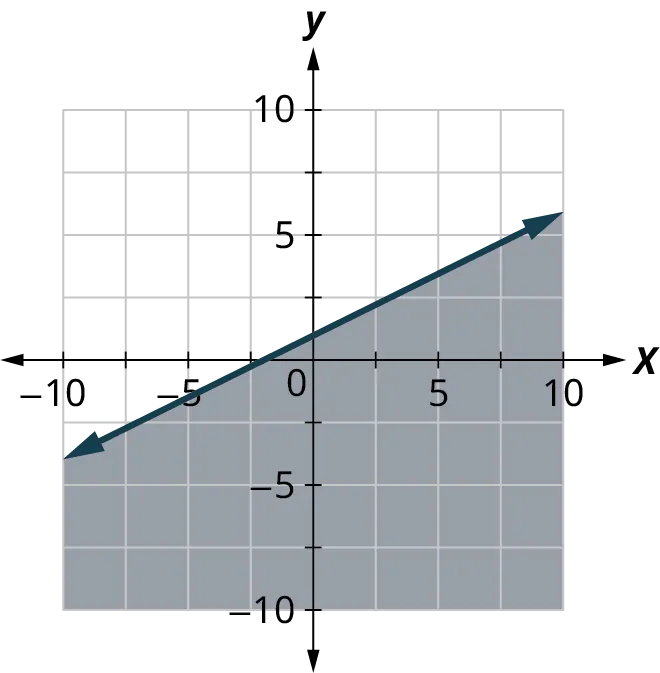29 .
Write the inequality shown by the graph with the boundary line $y = - \frac{1}{3}x - 2$.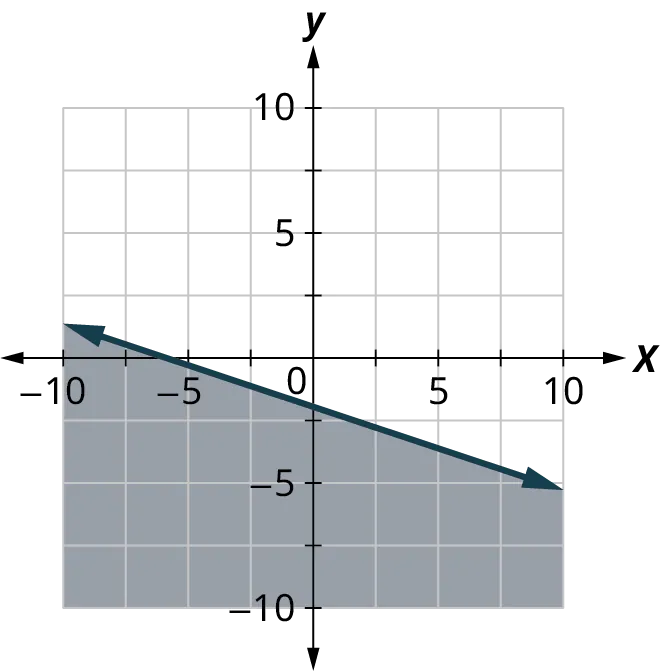30 .
Write the inequality shown by the shaded region in the graph with the boundary line $\;x + y = 5$.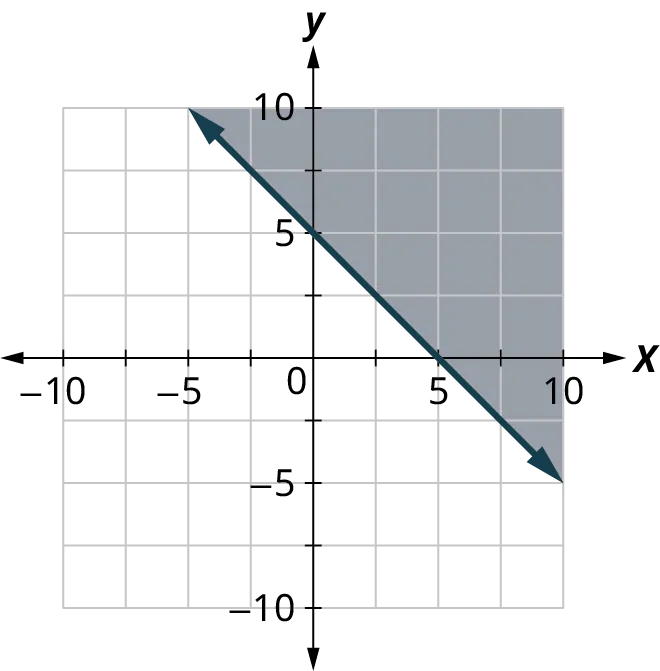31 .
Write the inequality shown by the shaded region in the graph with the boundary line $x + y = 3$.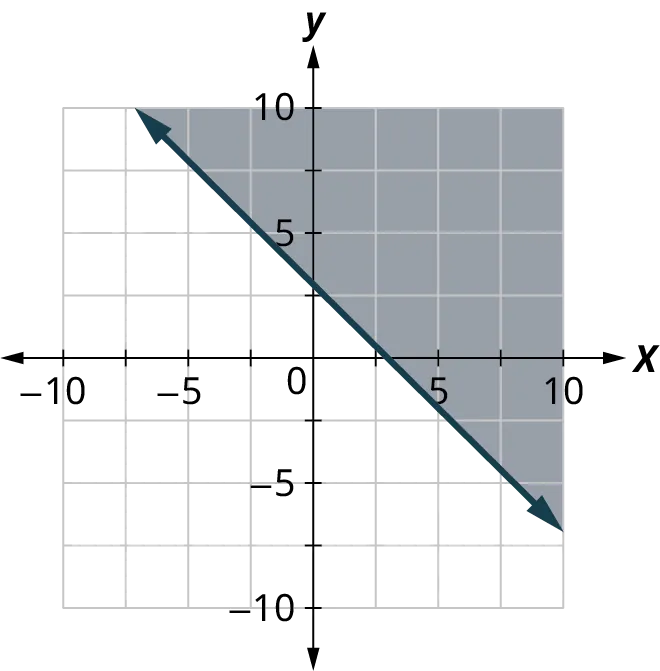32 .
Write the inequality shown by the shaded region in the graph with the boundary line $3x - y = 6$.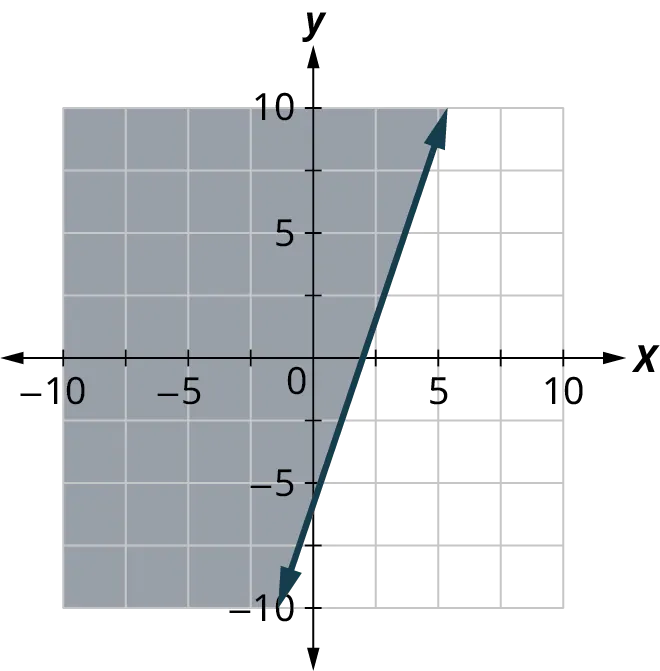33 .
Write the inequality shown by the shaded region in the graph with the boundary line $2x - y = 4$ .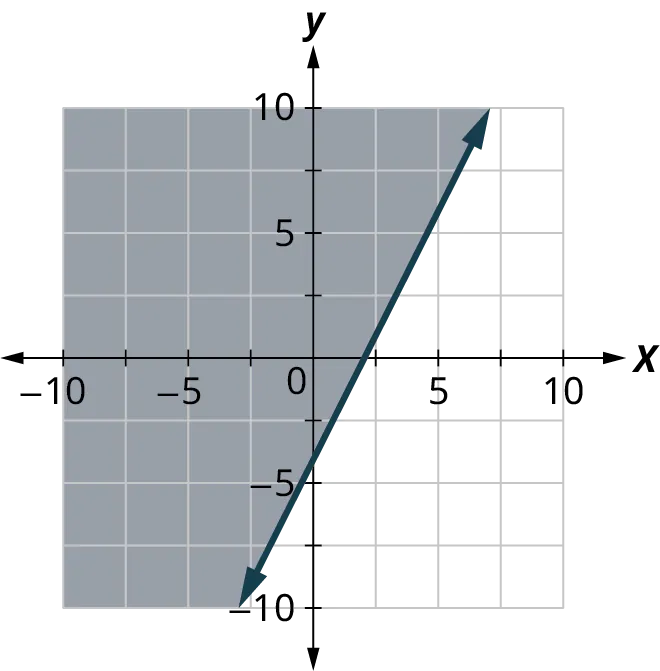For the following exercises, graph the linear inequality.
34 .
$y < \frac{3}{5}x + 2$
35 .
$y \leq - \frac{1}{2}x + 4$
36 .
$y \geq - \frac{1}{3}x - 2$
37 .
$x - y \leq 3$
38 .
$x - y \geq - 2$
39 .
$4x + y > - 4$
40 .
$x + 5y < - 5$
41 .
$3x + 2y \geq - 6$
42 .
$4x + 2y \geq - 8$
43 .
$y > 4x$
44 .
$y \leq - 3x$
Order a print copy

As an Amazon Associate we earn from qualifying purchases.